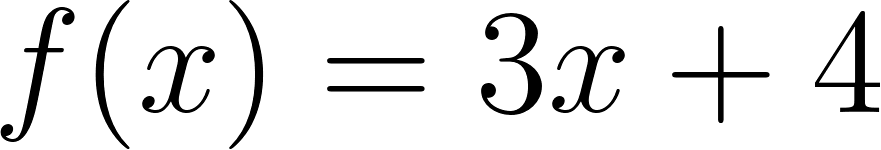×
Get Full Access to Calculus: Early Transcendentals - 1 Edition - Chapter 1.3 - Problem 13e
Get Full Access to Calculus: Early Transcendentals - 1 Edition - Chapter 1.3 - Problem 13e

×

# Where So inverses exist Use analytical and/or graphicalISBN: 9780321570567 2

## Solution for problem 13E Chapter 1.3

Calculus: Early Transcendentals | 1st Edition

• Textbook Solutions
• 2901 Step-by-step solutions solved by professors and subject experts
• Get 24/7 help from StudySoup virtual teaching assistantsCalculus: Early Transcendentals | 1st Edition

4 5 1 343 Reviews
13
2
Problem 13E

Where do inverses exist? Use analytical and/or graphical methods to determine the intervals on which the following functions have an inverse (make each interval as large as possible).Step-by-Step Solution:

Step 1 of 3

Given,Step 2 of 3

Step 3 of 3

##### ISBN: 9780321570567

This full solution covers the following key subjects: analytical, determine, exist, functions, graphical. This expansive textbook survival guide covers 85 chapters, and 5218 solutions. Calculus: Early Transcendentals was written by and is associated to the ISBN: 9780321570567. The answer to “?Where do inverses exist? Use analytical and/or graphical methods to determine the intervals on which the following functions have an inverse (make each interval as large as possible).” is broken down into a number of easy to follow steps, and 28 words. This textbook survival guide was created for the textbook: Calculus: Early Transcendentals, edition: 1. The full step-by-step solution to problem: 13E from chapter: 1.3 was answered by , our top Calculus solution expert on 03/03/17, 03:45PM. Since the solution to 13E from 1.3 chapter was answered, more than 388 students have viewed the full step-by-step answer.

Unlock Textbook Solution